Скачать презентацию Beginning the Problem Solving Process Tutorial 2 An

8bbfc5ee5f7b0a14b606d8271c50f49c.ppt

• Количество слайдов: 41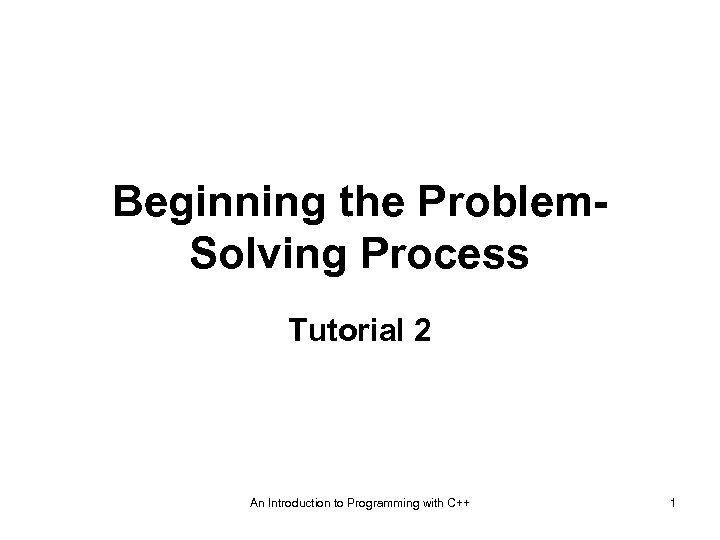Beginning the Problem. Solving Process Tutorial 2 An Introduction to Programming with C++ 1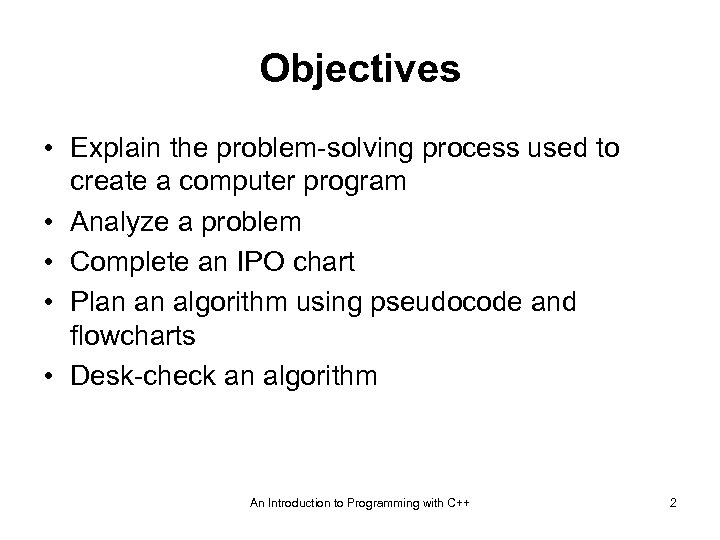Objectives • Explain the problem-solving process used to create a computer program • Analyze a problem • Complete an IPO chart • Plan an algorithm using pseudocode and flowcharts • Desk-check an algorithm An Introduction to Programming with C++ 2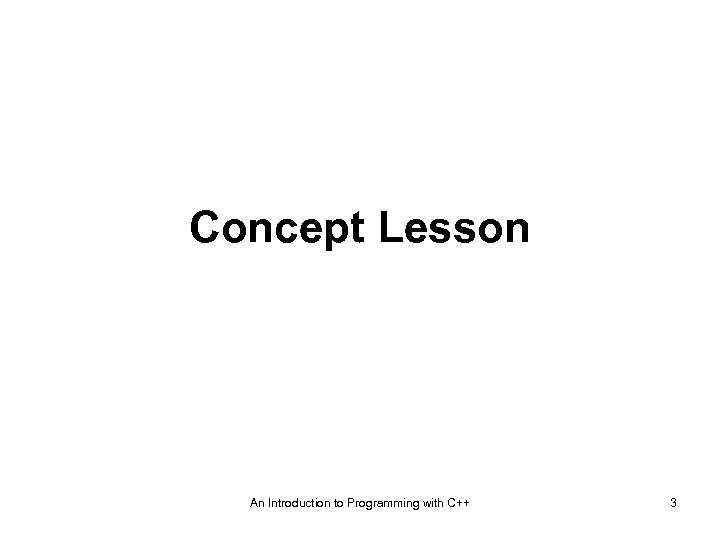Concept Lesson An Introduction to Programming with C++ 3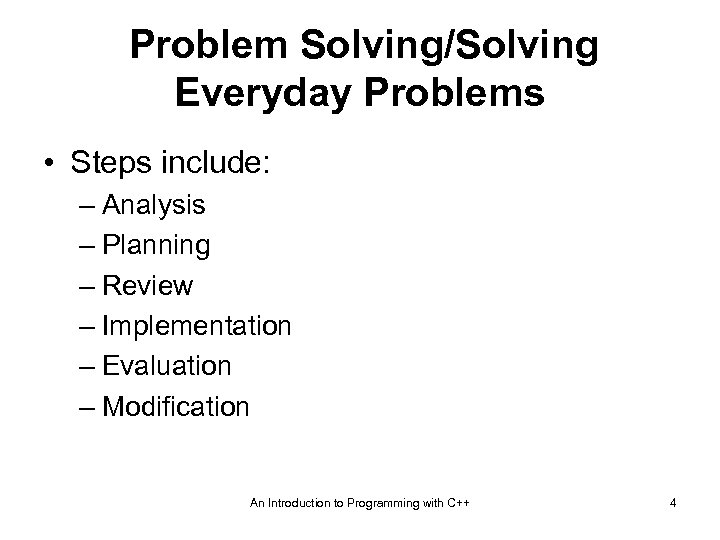Problem Solving/Solving Everyday Problems • Steps include: – Analysis – Planning – Review – Implementation – Evaluation – Modification An Introduction to Programming with C++ 4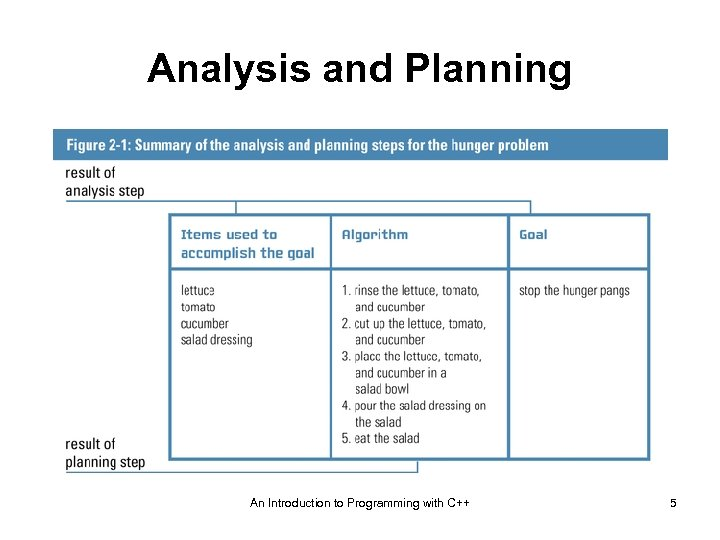Analysis and Planning An Introduction to Programming with C++ 5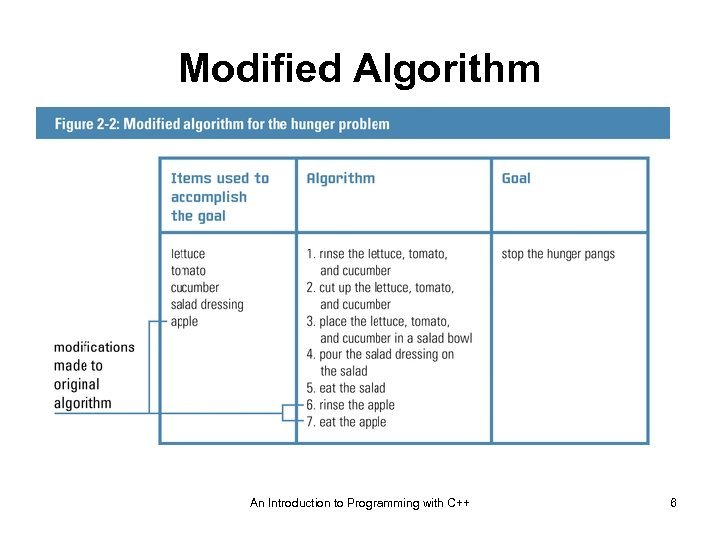Modified Algorithm An Introduction to Programming with C++ 6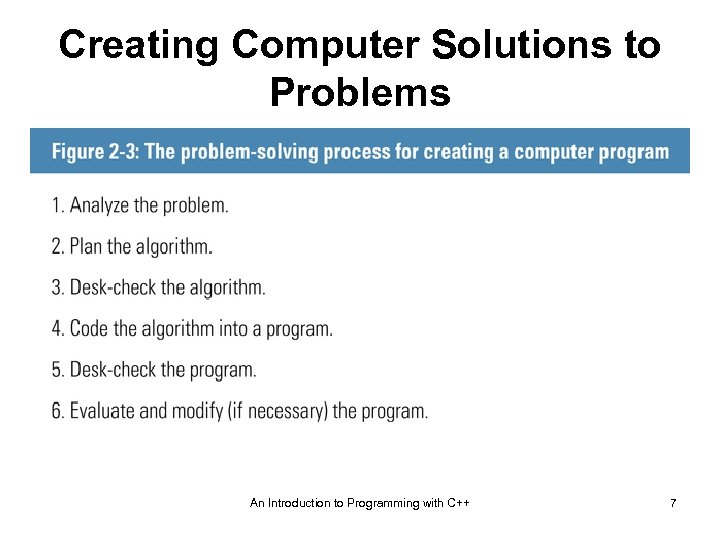Creating Computer Solutions to Problems An Introduction to Programming with C++ 7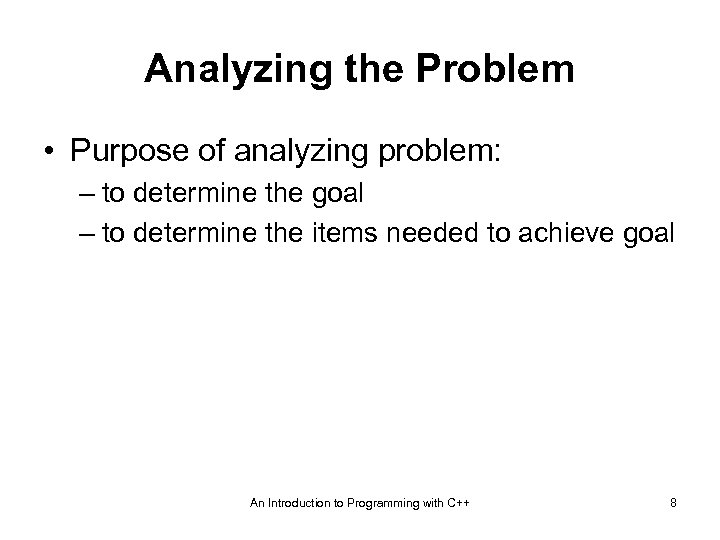Analyzing the Problem • Purpose of analyzing problem: – to determine the goal – to determine the items needed to achieve goal An Introduction to Programming with C++ 8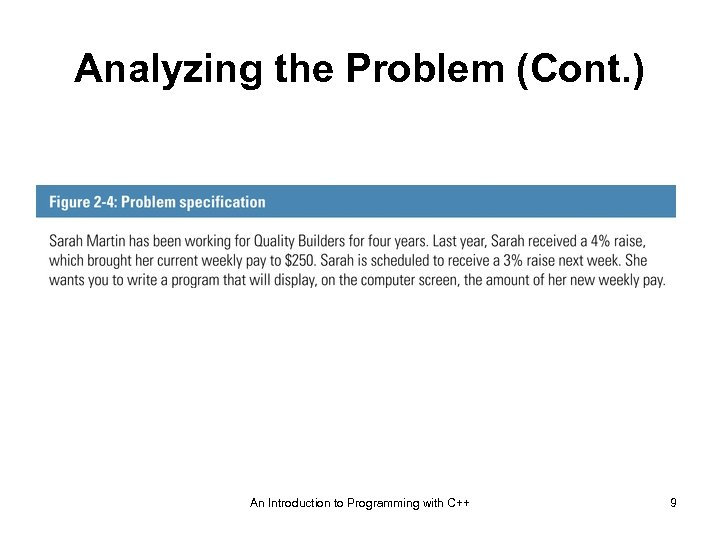Analyzing the Problem (Cont. ) An Introduction to Programming with C++ 9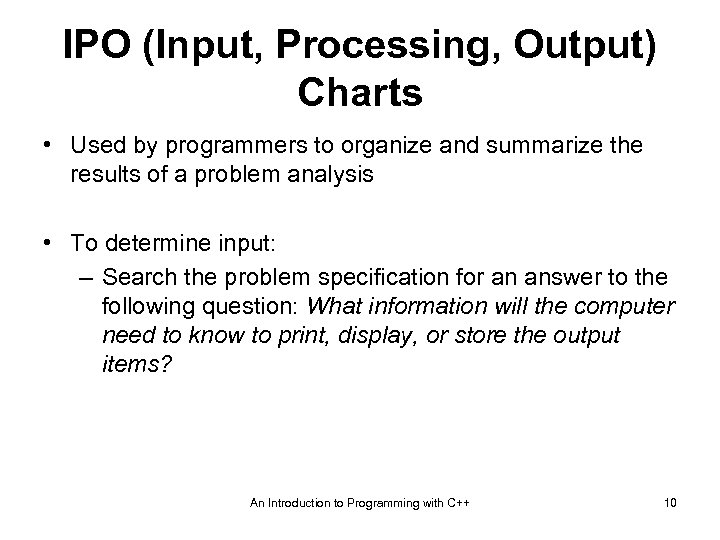IPO (Input, Processing, Output) Charts • Used by programmers to organize and summarize the results of a problem analysis • To determine input: – Search the problem specification for an answer to the following question: What information will the computer need to know to print, display, or store the output items? An Introduction to Programming with C++ 10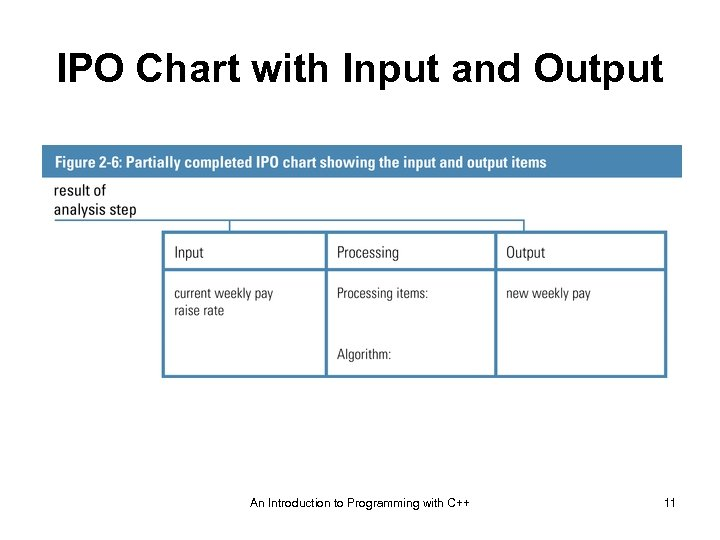IPO Chart with Input and Output An Introduction to Programming with C++ 11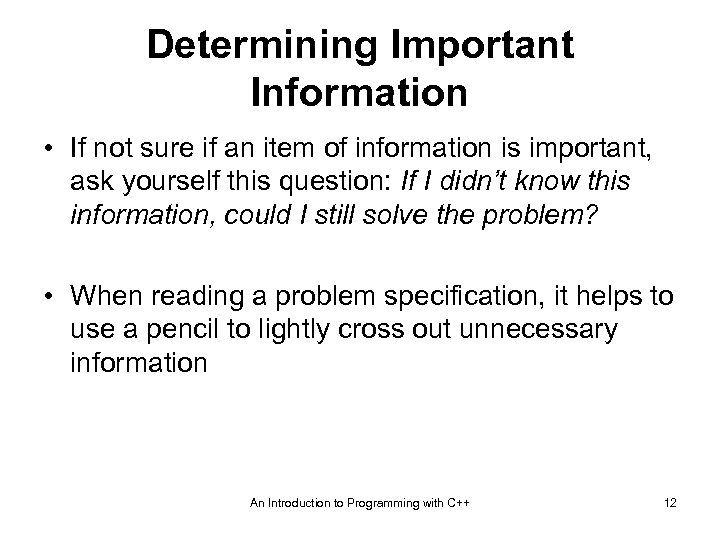Determining Important Information • If not sure if an item of information is important, ask yourself this question: If I didn’t know this information, could I still solve the problem? • When reading a problem specification, it helps to use a pencil to lightly cross out unnecessary information An Introduction to Programming with C++ 12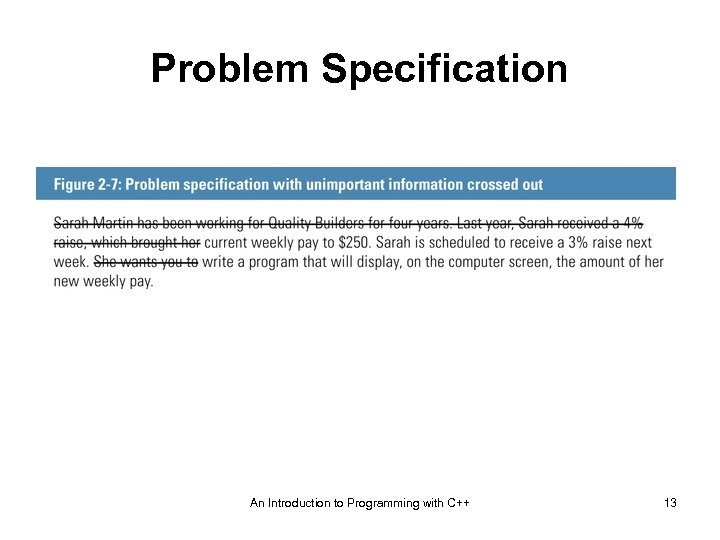Problem Specification An Introduction to Programming with C++ 13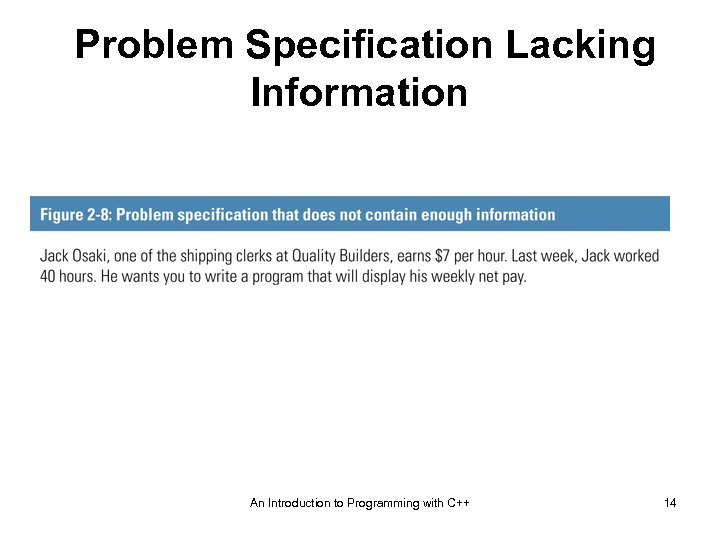Problem Specification Lacking Information An Introduction to Programming with C++ 14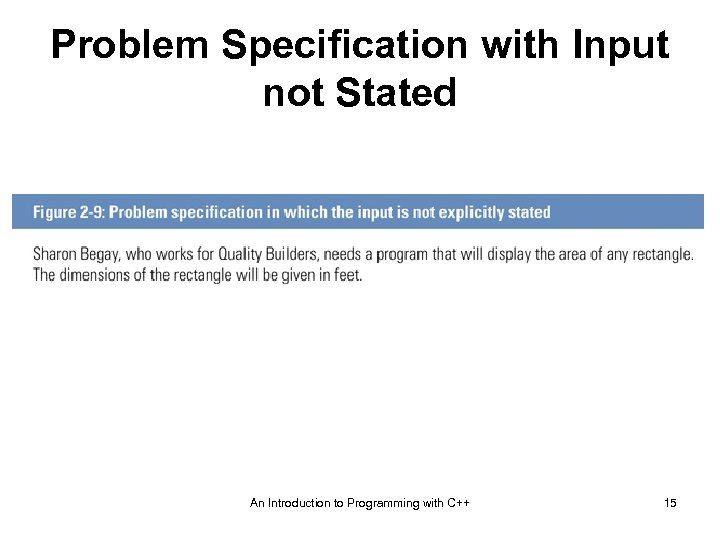Problem Specification with Input not Stated An Introduction to Programming with C++ 15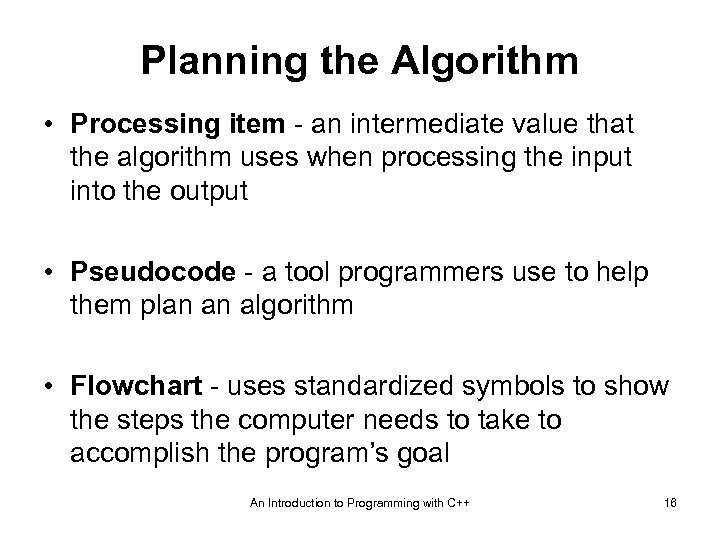Planning the Algorithm • Processing item - an intermediate value that the algorithm uses when processing the input into the output • Pseudocode - a tool programmers use to help them plan an algorithm • Flowchart - uses standardized symbols to show the steps the computer needs to take to accomplish the program’s goal An Introduction to Programming with C++ 16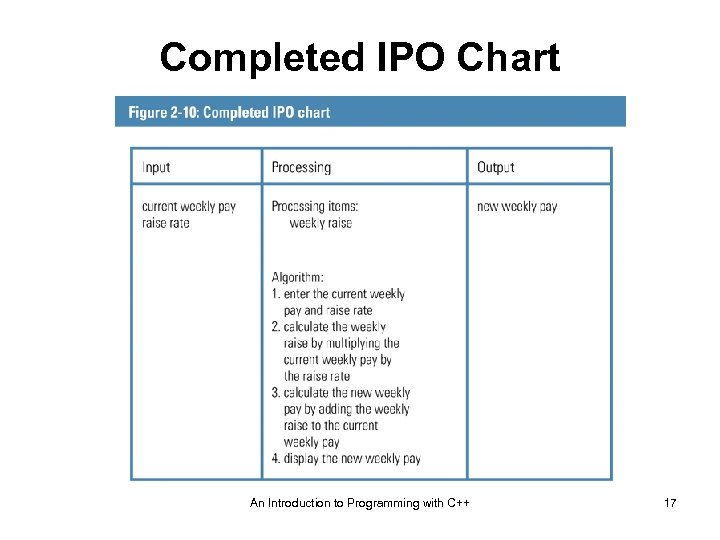Completed IPO Chart An Introduction to Programming with C++ 17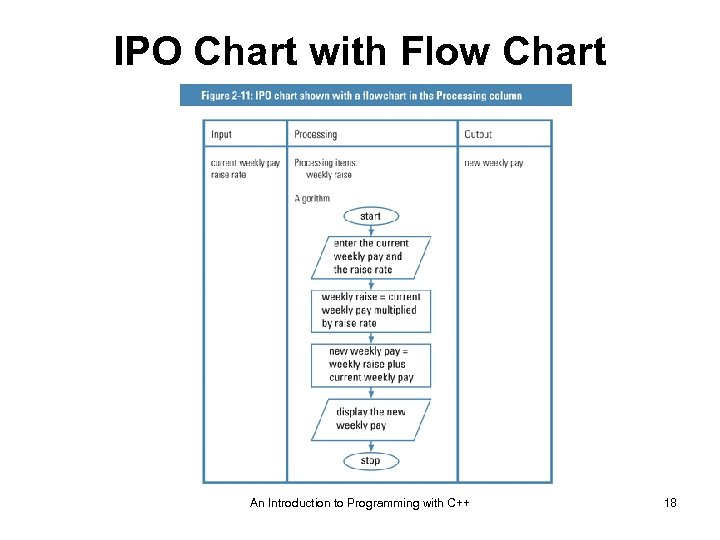IPO Chart with Flow Chart An Introduction to Programming with C++ 18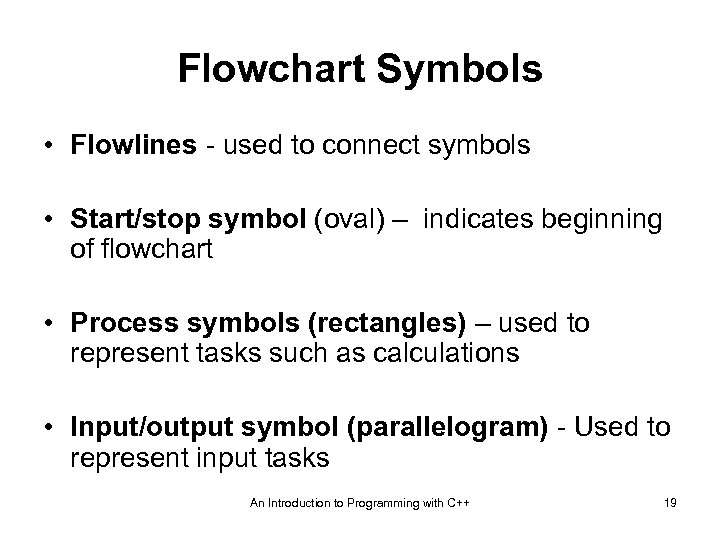Flowchart Symbols • Flowlines - used to connect symbols • Start/stop symbol (oval) – indicates beginning of flowchart • Process symbols (rectangles) – used to represent tasks such as calculations • Input/output symbol (parallelogram) - Used to represent input tasks An Introduction to Programming with C++ 19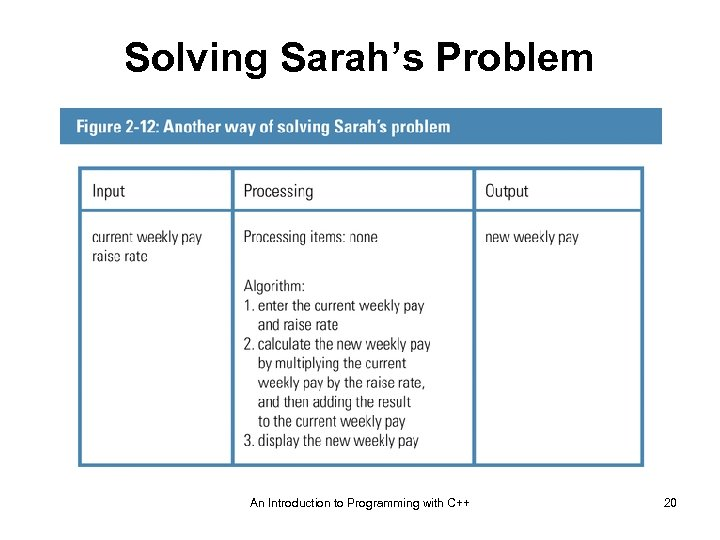Solving Sarah’s Problem An Introduction to Programming with C++ 20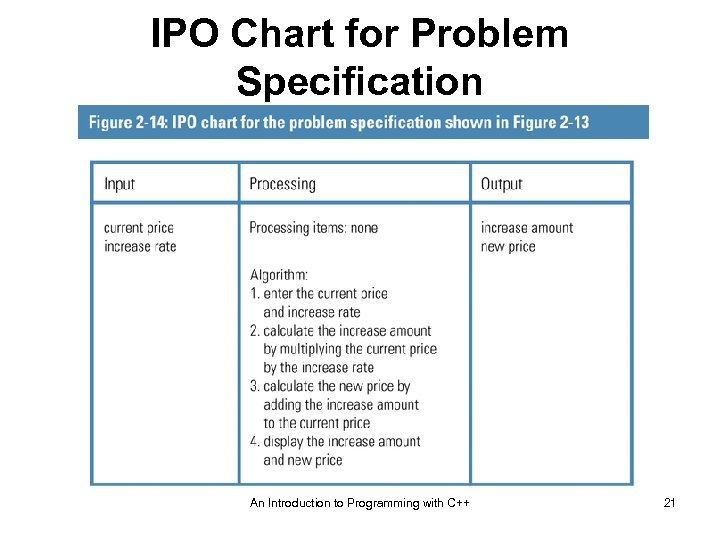IPO Chart for Problem Specification An Introduction to Programming with C++ 21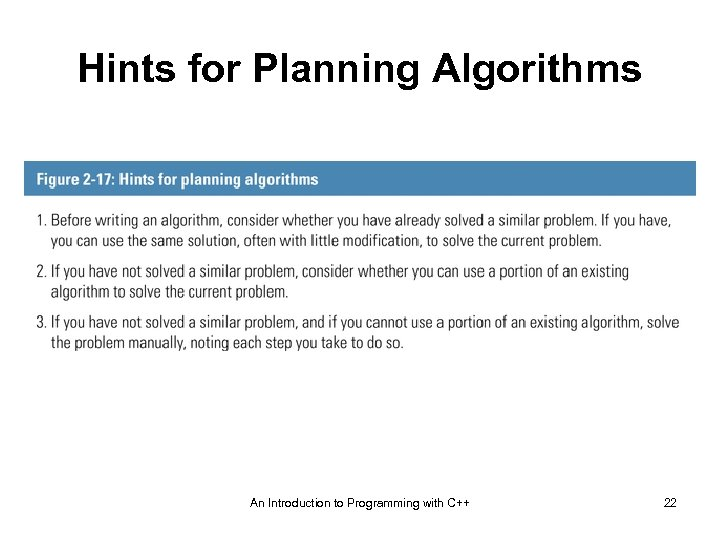Hints for Planning Algorithms An Introduction to Programming with C++ 22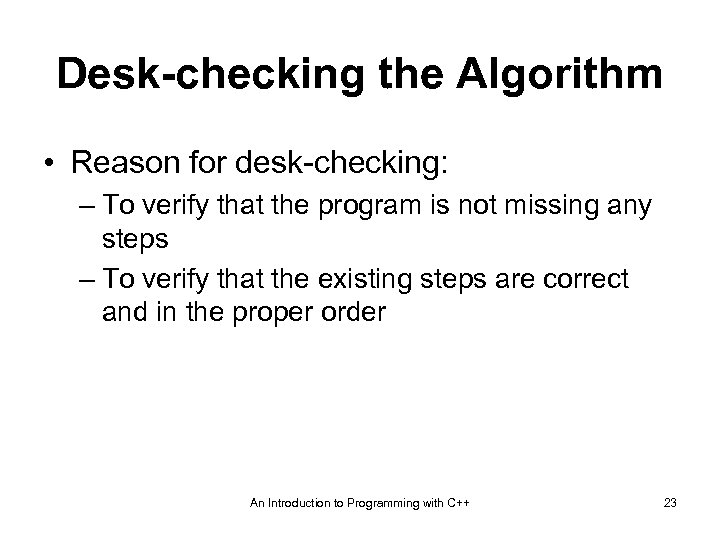Desk-checking the Algorithm • Reason for desk-checking: – To verify that the program is not missing any steps – To verify that the existing steps are correct and in the proper order An Introduction to Programming with C++ 23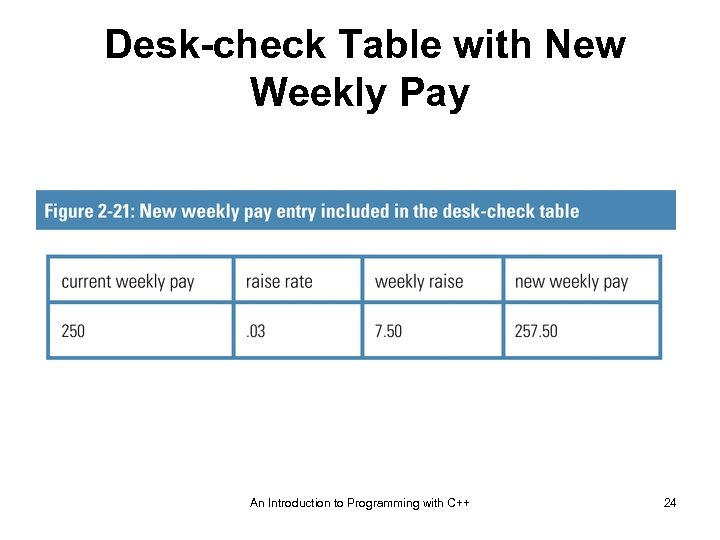Desk-check Table with New Weekly Pay An Introduction to Programming with C++ 24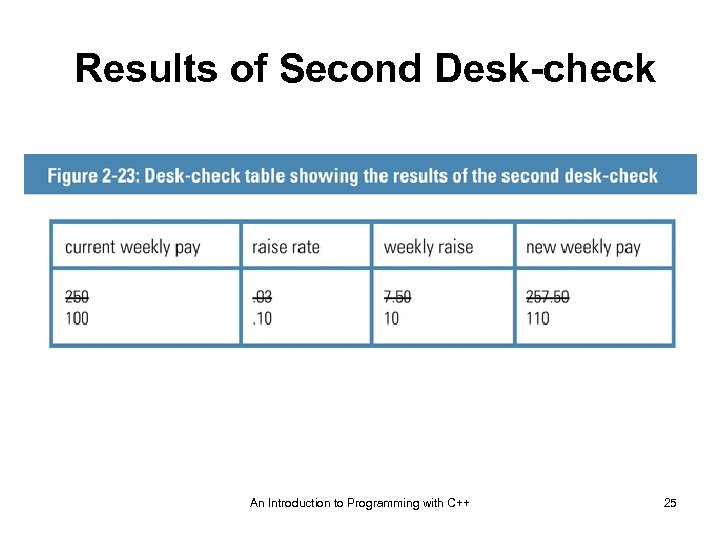Results of Second Desk-check An Introduction to Programming with C++ 25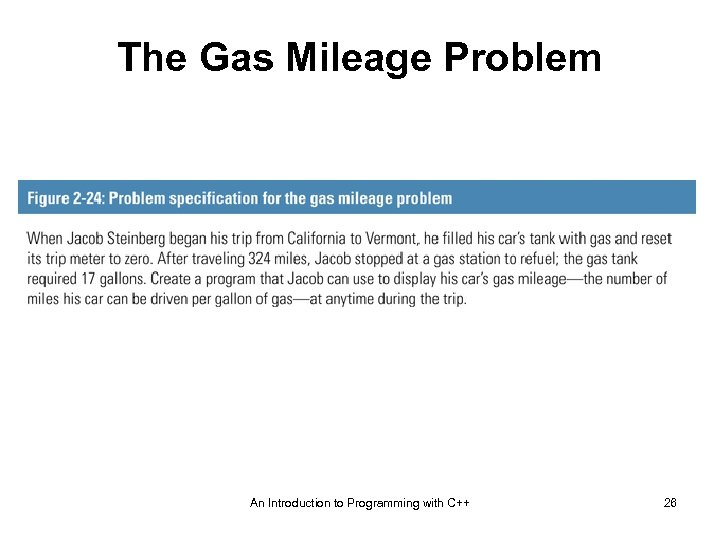The Gas Mileage Problem An Introduction to Programming with C++ 26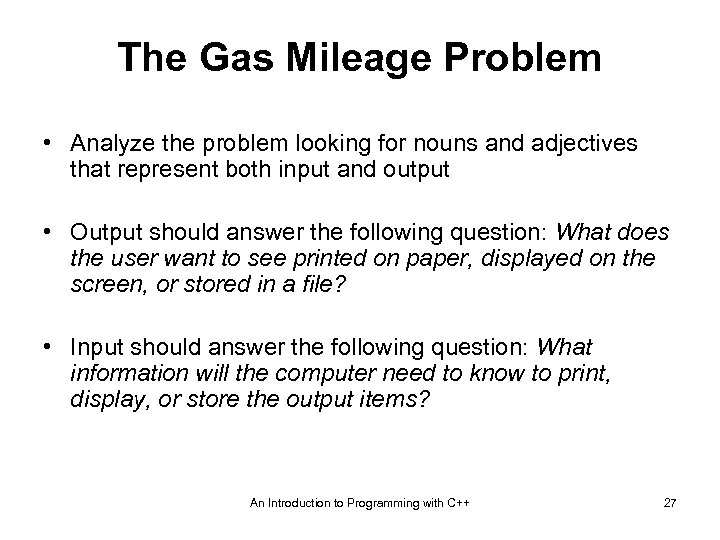The Gas Mileage Problem • Analyze the problem looking for nouns and adjectives that represent both input and output • Output should answer the following question: What does the user want to see printed on paper, displayed on the screen, or stored in a file? • Input should answer the following question: What information will the computer need to know to print, display, or store the output items? An Introduction to Programming with C++ 27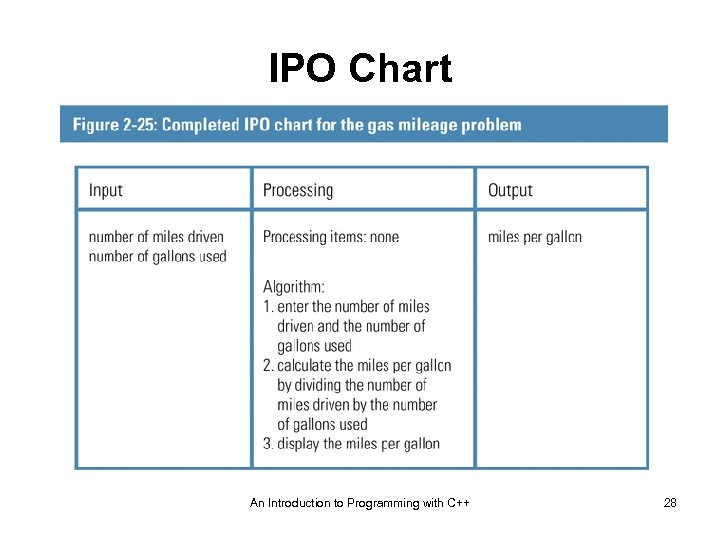IPO Chart An Introduction to Programming with C++ 28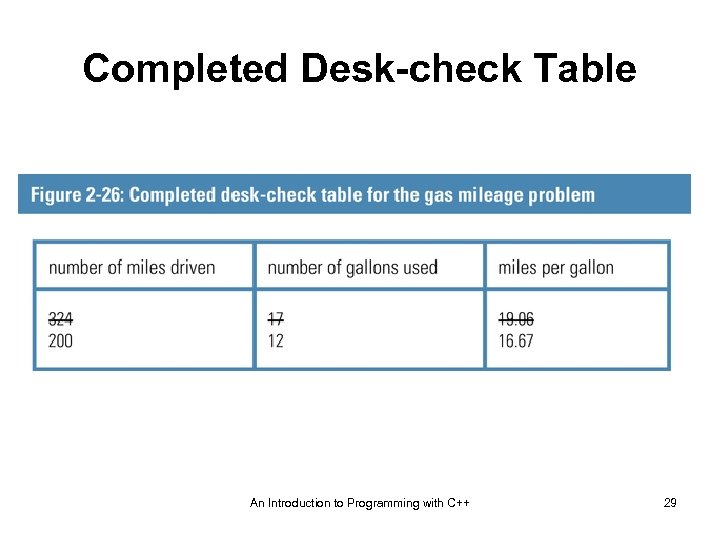Completed Desk-check Table An Introduction to Programming with C++ 29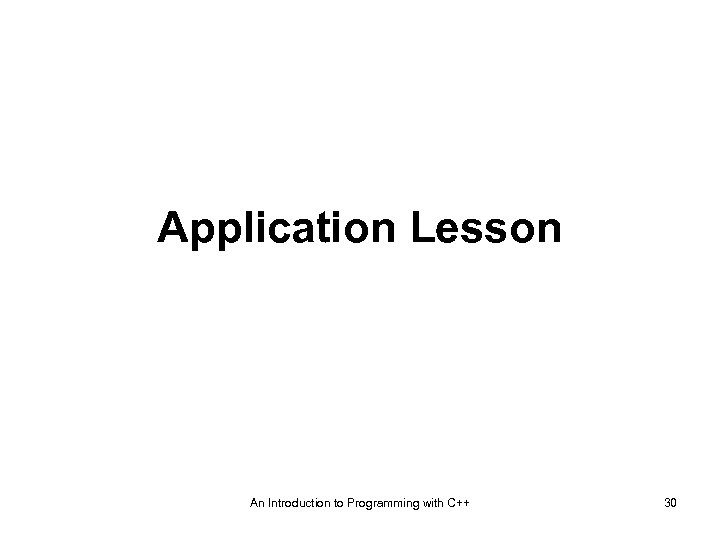Application Lesson An Introduction to Programming with C++ 30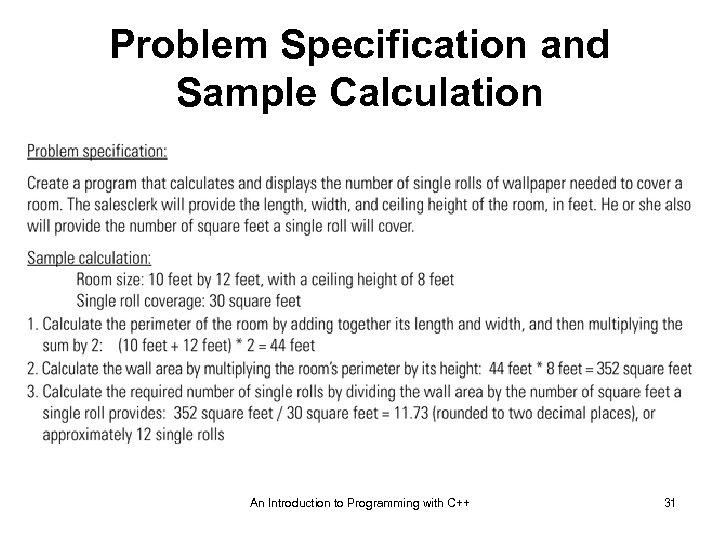Problem Specification and Sample Calculation An Introduction to Programming with C++ 31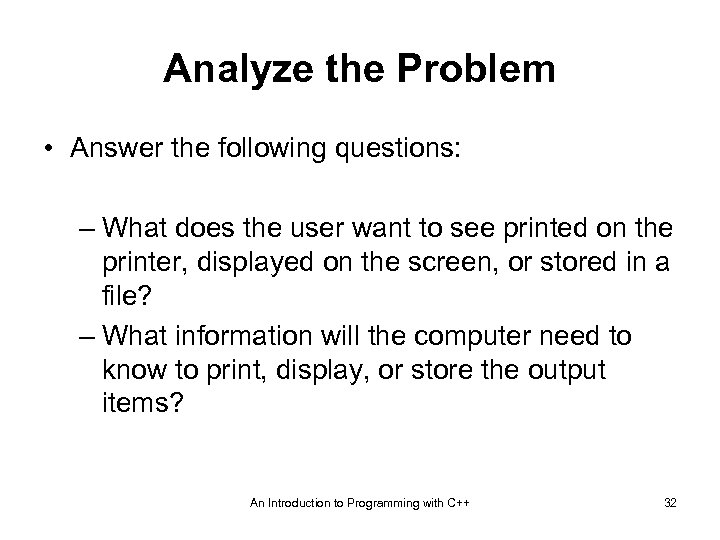Analyze the Problem • Answer the following questions: – What does the user want to see printed on the printer, displayed on the screen, or stored in a file? – What information will the computer need to know to print, display, or store the output items? An Introduction to Programming with C++ 32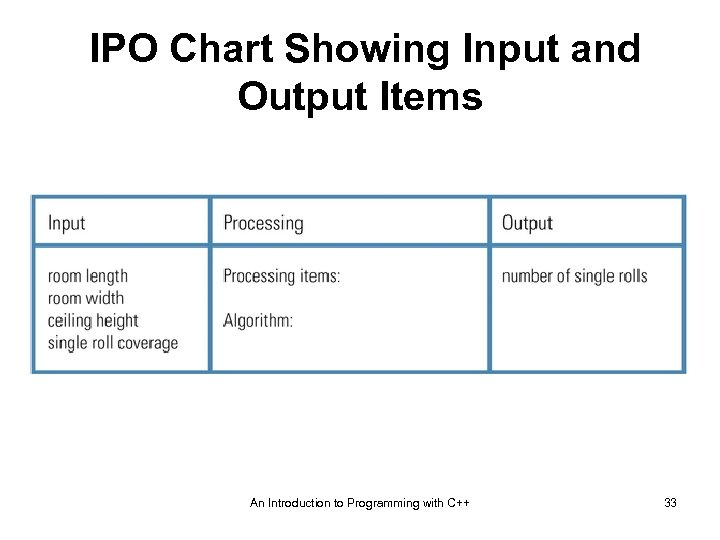IPO Chart Showing Input and Output Items An Introduction to Programming with C++ 33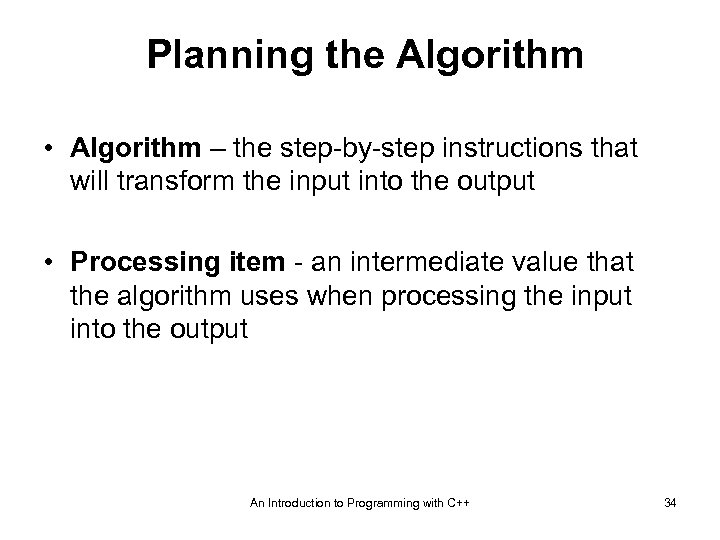Planning the Algorithm • Algorithm – the step-by-step instructions that will transform the input into the output • Processing item - an intermediate value that the algorithm uses when processing the input into the output An Introduction to Programming with C++ 34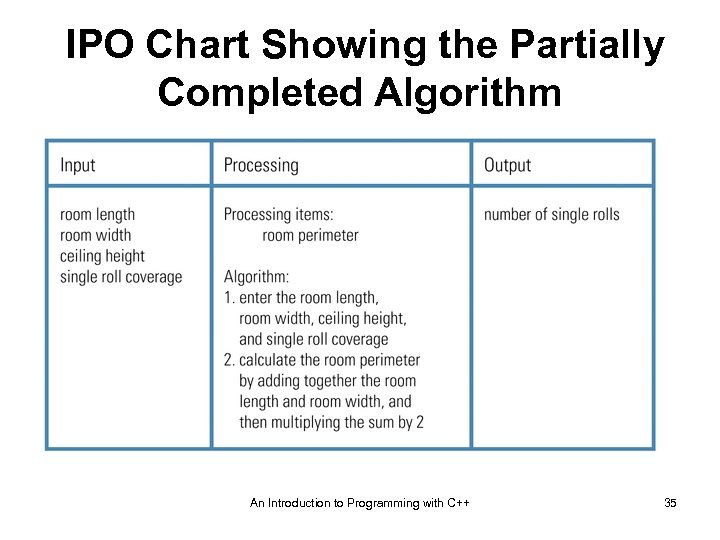IPO Chart Showing the Partially Completed Algorithm An Introduction to Programming with C++ 35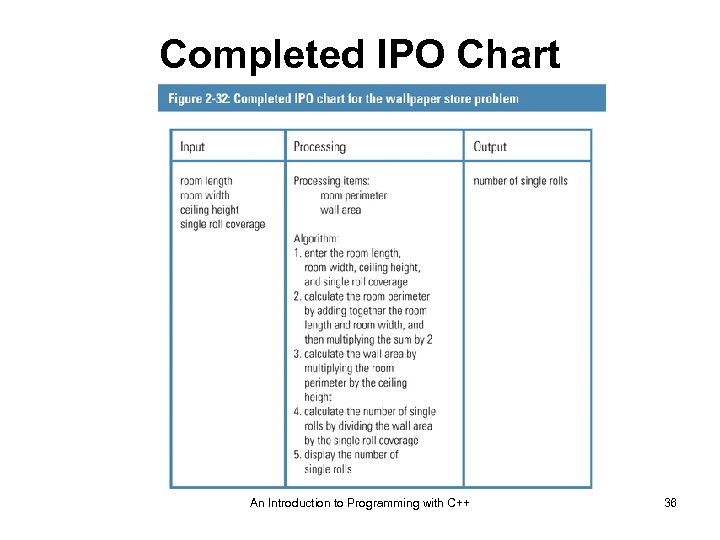Completed IPO Chart An Introduction to Programming with C++ 36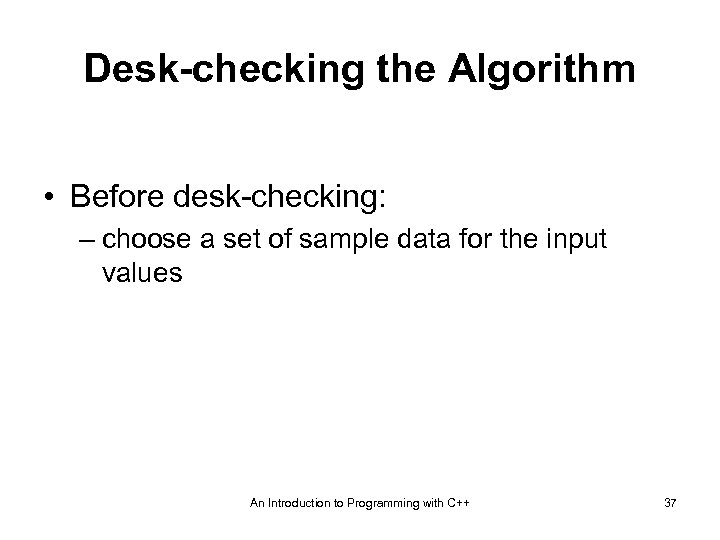Desk-checking the Algorithm • Before desk-checking: – choose a set of sample data for the input values An Introduction to Programming with C++ 37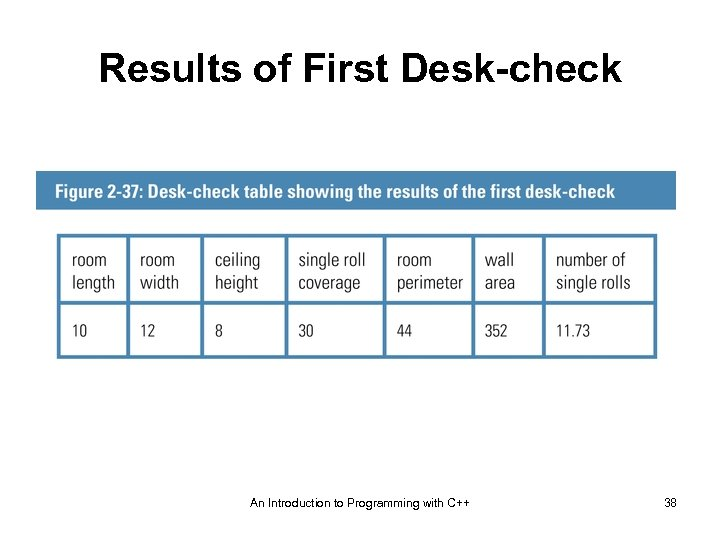Results of First Desk-check An Introduction to Programming with C++ 38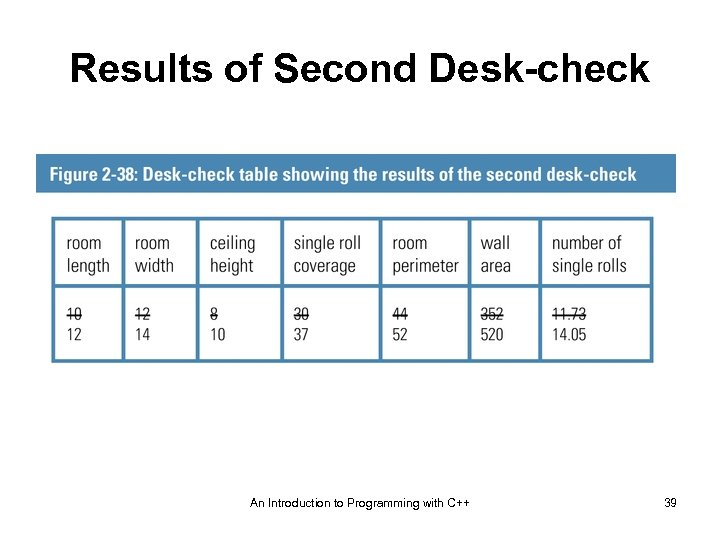Results of Second Desk-check An Introduction to Programming with C++ 39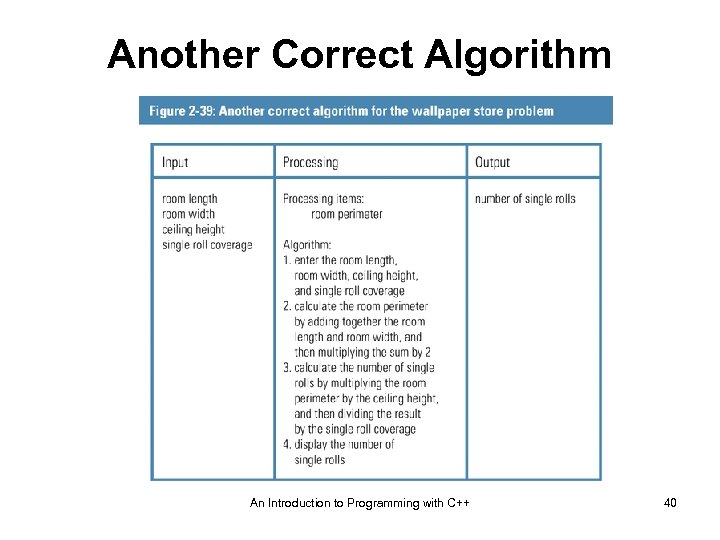Another Correct Algorithm An Introduction to Programming with C++ 40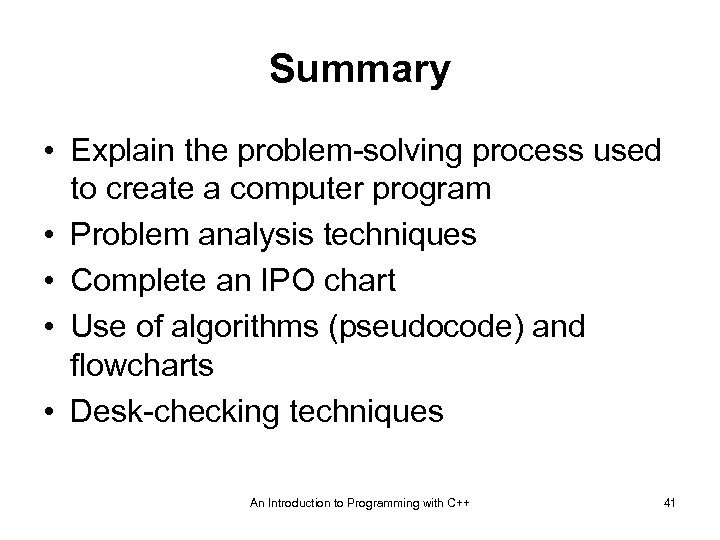Summary • Explain the problem-solving process used to create a computer program • Problem analysis techniques • Complete an IPO chart • Use of algorithms (pseudocode) and flowcharts • Desk-checking techniques An Introduction to Programming with C++ 41